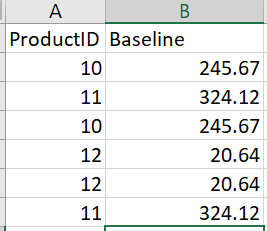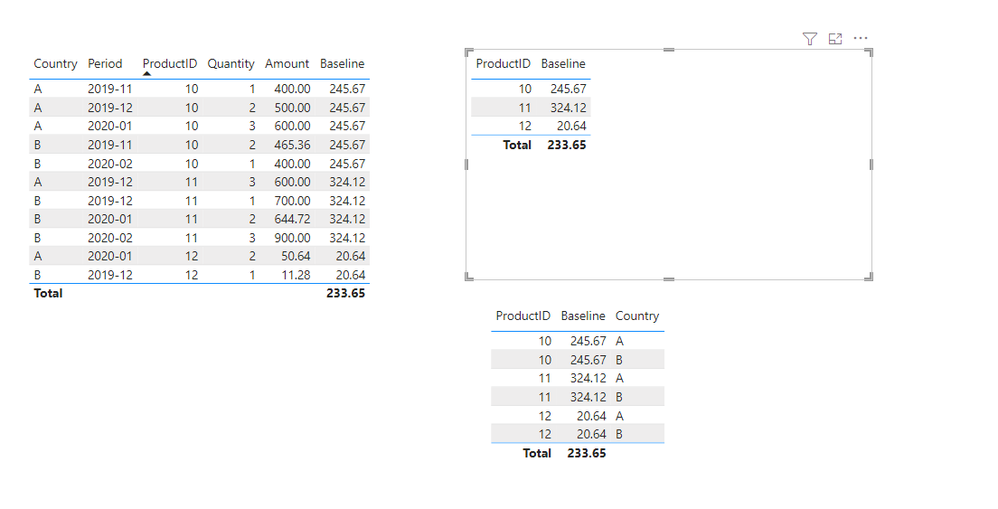cancel
Showing results for
Did you mean:Helper II

## Static Baseline Value (Grouped on Category ID)

I'm trying to Calculate a baseline value which calculates a products weighted price by calculating "SUM(Amount)/SUM(Quantity)" at a given point in time but the value must remain static for all items that are the same (regardless of additional columns or fields added).I've used a measure and have gotten the baseline value which give me correct values but doesn't remain static (as measure work with filter context) so I have tried adjusting the calculation to work with a column and it return incorrect values (since they work differently with row context) if someone could suggest a solution! my calculation is below when using the measure

Baseline =
Calculate ( DIVIDE (SUM ('Table'[Amount]), SUM ('Table'[Quantity]) ), 'Table'[Period] in {"2019 - 11", "2019 - 12", "2020 - 01"} )

if adding any other columns (fields) such as country or whatever the case is I need this value to stay static for only each product (in a sense like a group by "Product" in SQL if it helps any)

1 ACCEPTED SOLUTIONCommunity Support

Hi， @Euro0681

You should try ALLEXCEPT ,like this:

``````Baseline =
CALCULATE (
DIVIDE ( SUM ( 'Table'[Amount] ), SUM ( 'Table'[Quantity] ) ),
FILTER (
ALLEXCEPT ( 'Table', 'Table'[ProductID] ),
'Table'[_Period] IN { "2019 - 11", "2019 - 12", "2020 - 01" }
)
)``````

Please check the sample file for more details.Best Regards,
Community Support Team _ Eason

6 REPLIES 6Community Support

Hi， @Euro0681

You should try ALLEXCEPT ,like this:

``````Baseline =
CALCULATE (
DIVIDE ( SUM ( 'Table'[Amount] ), SUM ( 'Table'[Quantity] ) ),
FILTER (
ALLEXCEPT ( 'Table', 'Table'[ProductID] ),
'Table'[_Period] IN { "2019 - 11", "2019 - 12", "2020 - 01" }
)
)``````

Please check the sample file for more details.Best Regards,
Community Support Team _ EasonHelper II

@v-easonf-msft After Modifying the Calculation you provided I got it to work thanks! You can Ignore my previous replies. (Marked as solution)Helper II

@v-easonf-msft , when replicating this logic it gives me different values the moment i drag in a column that makes the products id repeat? idk if it has to do with the all except since product ID is also coming from product table (so i have 2 table (dimensions) Product and Date then i have my (fact) table connecting to both)Helper II

This is exactly what I'm needing but one question what if my Period column is coming from a different table than the product ID? (so for simplicity of my question i put them in the same table but i Have a date table where i'm pulling the period from so how would i adjust the all except logic?)Community Champion

try to add a ALL() argument, like this:

Baseline =
Calculate (
DIVIDE (SUM ('Table'[Amount]), SUM ('Table'[Quantity]) ),
'Table'[Period] in {"2019 - 11", "2019 - 12", "2020 - 01"},
ALL()
)Helper II

Didin't work as it only gives me one value thats static for all products and i need static values for each product (meaning product1 = 0.2 , product2 = 0.3 so anytime product1 is displayed it should show 0.2 and anytime product2 is shown it should show 0.3) by using your suggestiion i get one value like 8.2 for all products